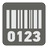CheckSum
Elementary
English ES

Errors during transcription are rather common. Unintentional keystrokes can cause characters to be rearranged, dropped or inserted in manually typed text.

Luckily for the robots, you have ways to solve problems like this. Your CheckSum helps to reduce the likelihood of errors by introducing a final character that is calculated from the previous characters. With the proper reasoning, the final character can always be calculated. This way, when inputs are entered, you can instantly verify that the final character matches the character predicted by CheckSum. If the two do not match, the input is rejected. The end result is that you would see fewer data entry errors.

### How CheckSum Works:

CheckSum reasoning will need map points. This is a definition lookup for how to process the input necessary to generate the final character, which will be enable us instantly verify that our input is correct. In other words, for each sequence character of the input, we are confident there can only be one possible input, all thanks to this final character.

The steps you must take to obtain the final character are as follows:
1. From the rightmost input, traverse from right to left, and apply 'map point character lookup' for even-indexed characters.
2. Add map point results for even-indexed characters with the unchanged digits from the original number.
3. Find the remainder of this sum with 10. For an example sum of 67, the remainder is 7. ( 67 modulo 10 = 7 )
4. Your final character should be 0 if the sum is a multiple-of-10, but it should be 10 minus remainder if the sum is not a multiple-of-10.
To generate the 'map point character lookup' table:
1. Double the value of a character.
2. If the product of this doubling operation is greater than 9 (e.g: 7 * 2 = 14), reduce it by summing the digits of the product. (i.e., find its digital root. e.g., 10: 1 + 0 = 1, 14: 1 + 4 = 5) Only do this once. Even if the sum is greater than 9, leave it alone.

### Could you give me an example please?

Okay, take a look this example for a (pretend) account number: "7992739871". We will add final character to it, so it looks something like: "7992739871x," with x being the final character-to-be-found.

Here is what a 'map point character lookup' table looks like for 0 through 9:

 Digits...

And here is how we will obtain final character:

 Index : 0 1 2 3 4 5 6 7 8 9 Reversed : 1 7 8 9 3 7 2 9 9 7 From table : 2 7 6 4 9 Total: 67 Unchanged : 7 9 7 9 7 Final Character : 10 - ( 67 % 10 ) = 10 - 7 = 3

So, we can release the account number as follows: 799 273 9871 3

### Alphanumeric Input

To make this more interesting, we can use alphanumeric input, which is a possible combination of 10 digits and 26 capital letters. It means that we will have to upgrade our map point to support letters. How we achieve that? We use each character's ASCII value to help us determine the character sequence. For example: 'A' has an ASCII value 65. To determine its sequence in our map, we need to substract 48.

For this example, 'A' is 7 since 65 - 48 = 17, 17 * 2 = 34 and 3 + 4 = 7. (Remember to only apply 'map point character lookup' to even-indexed characters. If 'A' is an odd-indexed character, its value is 17)

### Alphanumeric Example

Alright, we have another example here: "139MT". Let's see how we can obtain the final character...

 Reversed : T M 9 3 1 Sum of digits : 9 29 9 3 2 Total: 52 Final Character : 10 - ( 52 % 10 ) = 10 - 2 = 8

Here is detail for how we do it:

• T:  ASCII of 84, 84 - 48 = 36, 36 * 2 = 72, and 7 + 2 = 9
• M:  ASCII of 77, and 77 - 48 = 29
• 9:  from map point is, 9, or ASCII of 57, 57 - 48 = 9, 9 * 2 = 18, and 1 + 8 = 9
• 3:  just 3, or ASCII of 51, and 51 - 48 = 3
• 1:  from map point is, 2, or ASCII of 49, 49 - 48 = 1, and 1 * 2 = 2
• Sum of digits is 52, since 9 + 29 + 9 + 3 + 1 = 52
• Final character is 8, since 10 - ( 52 % 10 ) = 10 - 2 = 8

Now it's time to test your CheckSum module!

Input: Unsanitized numeric or alphanumeric due to formatting purpose

Output: List of its final character and sum of digits

Example:

```checkio("799 273 9871") == ["3", 67]
checkio("139-MT") == ["8", 52])
checkio("123") == ["0", 10])
checkio("999_999") == ["6", 54])
checkio("+61 820 9231 55") == ["3", 37])
checkio("VQ/WEWF/NY/8U") == ["9", 201])```
You should be an authorized user in order to see the full description and start solving this mission.